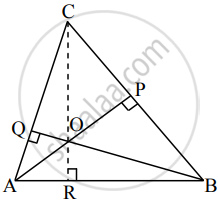# Prove that altitudes of a triangle are concurrent - Mathematics and Statistics

Sum

Prove that altitudes of a triangle are concurrent

#### Solution

Consider ∆ABC.

Let AP ⊥ BC and BQ ⊥ AC.

Let AP and BQ intersect at O.

Join OC and extend OC to meet AB at R.

To prove that CR is also the altitude of ∆ABC.

i.e., to prove that CR ⊥ ABLet bar"a", bar"b", bar"c" be the position vectors of the points A, B, C respectively.

Consider bar"AP" ⊥ bar"BC"

∴ bar"AO" ⊥ bar"BC"

∴ bar"AO" * bar"BC" = 0

∴ -bar"a"*(bar"c" - bar"b") = 0  .......[∵ bar"AO" = -bar"OA"]

∴ bar"a"*bar"c" - bar"a".bar"b" = 0    .......(i)

Now, bar"BQ" ⊥ bar"AC"

∴ bar"BO" ⊥ bar"AC"

∴ bar"BO" * bar"AC" = 0

∴ -bar"b"*(bar"c" - bar"a") = 0 .......[∵ bar"BO" = -bar"OB"]

∴ bar"b"*bar"c" - bar"b"*bar"a" = 0  .......(ii)

Comparing equations (i) and (ii), we get

∴ bar"a"*bar"c" - bar"a"*bar"b" = bar"b"*"c" - bar"b"*bar"a"

∴ bar"a"*bar"c" = bar"b"*bar"c"

∴ bar"a"*bar"c" - bar"b"*bar"c" = 0

∴ bar"c"*(bar"a" - bar"b") = 0

∴ -bar"c"*(bar"a" - bar"b") = 0

∴ bar"CO" ⊥ bar"BA"

∴ bar"CR" ⊥ bar"BA"

∴ CR ⊥ BA

∴ CR is also the altitude of ∆ABC.

∴ AP, BQ, CR intersect at O.

∴ All three altitudes of ∆ABC intersect at a common point.

Thus, the altitudes of a triangle are concurrent.

Concept: Section Formula
Is there an error in this question or solution?